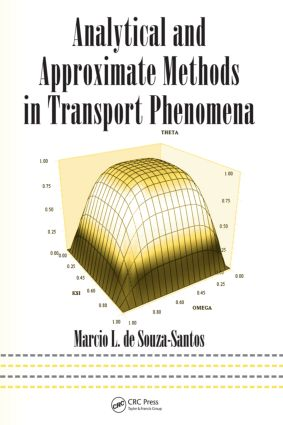Analytical and Approximate Methods in Transport Phenomena

1st Edition

CRC Press

592 pages | 196 B/W Illus.

Purchasing Options:\$ = USD
Hardback: 9780849334085
pub: 2007-10-29
SAVE ~\$43.00
\$215.00
\$172.00
x
eBook (VitalSource) : 9780429135590
pub: 2007-10-29
from \$28.98

FREE Standard Shipping!

Description

On the job or in the field, when facing a problem with differential equations and boundary conditions, most likely you don’t have time to read through several publications in search of a method that may or may not solve your problem. Organized for quick and easy access to practical solutions, Analytical and Approximate Methods in Transport Phenomena is a reference for the day-to-day problems encountered when working with variables in heat, mass, or momentum transfer.

This text is organized differently from usual resources on applied mathematics for engineers. First, it introduces a new classification system of the problem based on just three numbers, so locating the appropriate solution method is quick and easy. Second, the author presents mathematical methods with applications in mind, introducing examples, as well as common or possible solutions, before presenting any mathematical theory or method. This allows you to identify the issue you need to resolve, then apply the appropriate method to the problem at hand. The book also includes practical discussions of the consequences and applications of various solutions.

The book highlights mathematical methods as tools for solving practical problems, not as a primary objective. Its structure and focus on application, with just the right amount of mathematical rigor, makes it the most effective manual available for easily finding the analytical methods needed to solve transport problems.

Problems 111; One Variable, 1st Order, 1st Kind Boundary Condition

Introduction

Heating of a Solid

Flow between Two Drums

Heating of a Fluid in a Stirring Tank

Heated Batch Reactor

Reactor with a Time-Controlled Rate

Pressure in a Resting Fluid

Pressure in Fluid under Rotational Movement

Plug-Flow Reactor

Heat Conduction in an Indefinite Wall

Plate-and-Cone Viscometer

Thermocouple

Exercises

References

Problems 112; One Variable, 1st Order, 2nd Kind Boundary Condition

Introduction

Heating of a Solid

Heat Conduction in a Spherical Shell

Batch Reactor

Plug-Flow Reactor

Exercises

Reference

Problems 113; One Variable, 1st Order, 3rd Kind Boundary Condition

Introduction

Heating of a Solid with Controlled Heat Transfer Rate

Temperature-Controlled Batch Reactor

Batch Reactor

Exercises

Problems 121; One Variable, 2nd Order, 1st Kind Boundary Condition

Introduction

Mass Transfer through a Cylindrical Rod

Mass Transfer in a Rod with Variable Diffusivity

Conduction through a Pipe Wall

Electrically Heated Pipe Wall

Heat Transfer in a Spherical Shell

Absorption without Reaction

Diffusion through a Spherical Shell with Zero-Order Reaction

Absorption with Homogeneous Reaction

Reacting Particle

Heat Transfer through a Reacting Plate

Exercises

References

Problems 122; One Variable, 2nd Order, 2nd Kind Boundary Condition

Introduction

Flow on an Inclined Plate

Flow in an Inclined Tube

Rectangular Fin

Circular Fin

Film Condensation

Heat Transfer through a Reacting Plate

Exercises

References

Problems 123; One Variable, 2nd Order, 3rd Kind Boundary Condition

Introduction

Heat Transfer between a Plate and Fluids

Heat Transfer in a Spherical Shell

Reacting Particle

Heat Transfer between a Reacting Plate and a Fluid

Exercises

References

Problems 211; Two Variables, 1st Order, 1st Kind Boundary

Condition

Introduction

Pressure in Fluid Under Rotational Movement

Heating a Flowing Liquid

Plug-Flow Reactor

Exercises

Problems 212; Two Variables, 1st Order, 2nd Kind Boundary Condition

Introduction

Heating of Flowing Liquid

Plug-Flow Reactor

Exercises

Problems 213; Two Variables, 1st Order, 3rd Kind Boundary Condition

Introduction

Heating of Flowing Liquid

Dynamic Plug-Flow Reactor

Exercises

Problems 221; Two Variables, 2nd Order, 1st Kind Boundary Condition

Introduction

Heating an Insulated Rod or a Semi-Infinite Body

Sudden Motion of a Plate

Heating a Flowing Liquid

Plug-Flow Reactor

Temperature Profile in a Rectangular Plate

Heating a Liquid Film

Absorbing Flowing Film

Exercises

Reference

Problems 222; Two variables, 2nd Order, 2nd Kind Boundary Condition

Introduction

Heating an Insulated Rod or Semi-Infinite Body

Drying of a Spherical Particle

Heating a Cylinder

Insulated Rod with Prescribed Initial Temperature Profile

Plate-and-Cone Viscometer

Heating a Rectangular Plate

Exercises

References

Problems 223; Two Variables, 2nd Order, 3rd Kind Boundary Condition

Introduction

Convective Heating of an Insulated Rod or Semi-Infinite Body

Drying of a Spherical Particle

Convective Heating of a Cylinder

Convective Heating of Insulated Rod with Prescribed Temperature at One End

Convective Heating of a Plate

Convective Heating of a Plate with Prescribed

Temperature Function at One Face

Exercises

References

Problems 311; Three Variables, 1st Order, 1st Kind Boundary Condition

Introduction

Heating a Flowing Liquid

Two-Dimensional Reacting Flow

Exercises

Problems 312; Three Variables, 1st Order, 2nd Kind Boundary Condition

Introduction

Heating a Flowing Liquid

Two-Dimensional Reacting Flow

Exercises

Problems 313; Three Variables, 1st Order, 3rd Kind Boundary Condition

Introduction

Heating a Flowing Liquid

Two-Dimensional Reacting Flow

Exercises

Problems 321; Three Variables, 2nd Order, 1st Kind Boundary Condition

Introduction

Temperatures in a Rectangular Plate

Exercises

Appendix A: Fundamental Equations of Transport Phenomena

Appendix B: Fundamental Aspects of Ordinary Differential Equations

Appendix C: Method of Power Series and Special Functions

Appendix D: Laplace Transform

Appendix E: Method of Weighted Residuals

Appendix F: Method of Similarity

Appendix G: Fourier Series and Method of Separation of Variables

Appendix H: Fourier Transforms

Appendix I: Generalized Representation by Series

Exercises

References

Index

de Souza-Santos\, Marcio L.